# Golden RatioThe golden ratio (symbol is the Greek letter "phi" shown at left) is a special number approximately equal to 1.618 It appears many times in geometry, art, architecture and other areas.

## The Idea Behind It

 We find the golden ratio when we divide a line into two parts so that: the whole length divided by the long part is also equal to the long part divided by the short partHave a try yourself (use the slider):

## BeautyThis rectangle has been made using the Golden Ratio, Looks like a typical frame for a painting, doesn't it?

Some artists and architects believe the Golden Ratio makes the most pleasing and beautiful shape.

Do you think it is the "most pleasing rectangle"?

Maybe you do or don't, that is up to you!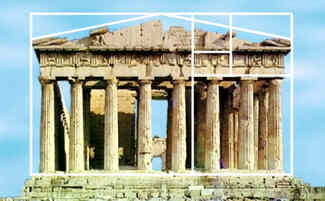Many buildings and artworks have the Golden Ratio in them, such as the Parthenon in Greece, but it is not really known if it was designed that way.

## The Actual Value

The Golden Ratio is equal to:

1.61803398874989484820... (etc.)

The digits just keep on going, with no pattern. In fact the Golden Ratio is known to be an Irrational Number, and I will tell you more about it later.

## Calculating It

You can calculate it yourself by starting with any number and following these steps:

• A) divide 1 by your number (=1/number)
• B) add 1
• C) that is your new number, start again at A

With a calculator, just keep pressing "1/x", "+", "1", "=", around and around. I started with 2 and got this:

Number 1/Number Add 1
2 1/2=0.5 0.5+1=1.5
1.5 1/1.5 = 0.666... 0.666... + 1 = 1.666...
1.666... 1/1.666... = 0.6 0.6 + 1 = 1.6
1.6 1/1.6 = 0.625 0.625 + 1 = 1.625
1.625 1/1.625 = 0.6154... 0.6154... + 1 = 1.6154...
1.6154...

It is getting closer and closer!

But it takes a long time to get even close, but there are better ways and it can be calculated to thousands of decimal places quite quickly.

## Drawing ItHere is one way to draw a rectangle with the Golden Ratio:

• Draw a square (of size "1")
• Place a dot half way along one side
• Draw a line from that point to an opposite corner (it is √5/2 in length)
• Turn that line so that it runs along the square's side

Then you can extend the square to be a rectangle with the Golden Ratio.

## The Formula

That rectangle above shows us a simple formula for the Golden Ratio.

When one side is 1, the other side is: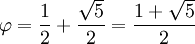The square root of 5 is approximately 2.236068, so the Golden Ratio is approximately (1+2.236068)/2 = 3.236068/2 = 1.618034. This is an easy way to calculate it when you need it.

Interesting fact: the Golden Ratio is also equal to 2 × sin(54°), get your calculator and check!

## Fibonacci Sequence

There is a special relationship between the Golden Ratio and the Fibonacci Sequence:

0, 1, 1, 2, 3, 5, 8, 13, 21, 34, ...

(The next number is found by adding up the two numbers before it.)

And here is a surprise: when we take any two successive (one after the other) Fibonacci Numbers, their ratio is very close to the Golden Ratio.

In fact, the bigger the pair of Fibonacci Numbers, the closer the approximation. Let us try a few:

A
B
B/A
2
3
1.5
3
5
1.666666666...
5
8
1.6
8
13
1.625
...
...
...
144
233
1.618055556...
233
377
1.618025751...
...
...
...

We don't even have to start with 2 and 3, here I chose 192 and 16 (and got the sequence 192, 16, 208, 224, 432, 656, 1088, 1744, 2832, 4576, 7408, 11984, 19392, 31376, ...):

A
B

B / A
192
16
0.08333333...
16
208
13
208
224
1.07692308...
224
432
1.92857143...
...
...
...
7408
11984
1.61771058...
11984
19392
1.61815754...
...
...
...

## The Most Irrational ...

I believe the Golden Ratio is the most irrational number. Here is why ...

 One of the special properties of the Golden Ratio is that it can be defined in terms of itself, like this: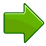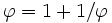(In numbers: 1.61803... = 1 + 1/1.61803...) That can be expanded into this fraction that goes on for ever (called a "continued fraction"):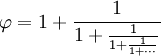So, it neatly slips in between simple fractions.

But many other irrational numbers are reasonably close to rational numbers (such as Pi = 3.141592654... is pretty close to 22/7 = 3.1428571...)## Pentagram

No, not witchcraft! The pentagram is more famous as a magical or holy symbol. And it has the Golden Ratio in it:

• a/b = 1.618...
• b/c = 1.618...
• c/d = 1.618...

Read more at Pentagram.

## Other Names

The Golden Ratio is also sometimes called the golden section, golden mean, golden number, divine proportion, divine section and golden proportion.